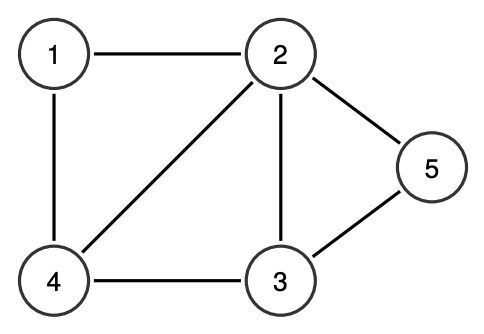시간 제한메모리 제한제출정답맞힌 사람정답 비율
0.5 초 512 MB160857553.571%

## 문제

Let G = (V, E) be a simple undirected graph with N vertices and M edges, where V = {1, . . . , N}. A tuple <u, v, w> is called as boomerang in G if and only if {(u, v),(v, w)} ⊆ E and u ≠ w; in other words, a boomerang consists of two edges which share a common vertex.

Given G, your task is to find as many disjoint boomerangs as possible in G. A set S contains disjoint boomerangs if and only if each edge in G only appears at most once (in one boomerang) in S. You may output any valid disjoint boomerangs, but the number of disjoint boomerangs should be maximum.

For example, consider a graph G = (V, E) of N = 5 vertices and M = 7 edges where E = {(1, 2), (1, 4), (2, 3), (2, 4), (2, 5), (3, 4), (3, 5)}.The maximum number of disjoint boomerangs in this example graph is 3. One example set containing the 3 disjoint boomerangs is {<4, 1, 2>,<4, 3, 2>,<2, 5, 3>}; no set can contain more than 3 disjoint boomerangs in this example.

## 입력

Input begins with a line containing two integers: N M (1 ≤ N, M ≤ 100000), representing the number of vertices and the number edges in G, respectively. The next M lines, each contains two integers: ui vi (1 ≤ ui < vi ≤ N), representing the edge (ui, vi) in G. You may safely assume that each edge appears at most once in the given list.

## 출력

The first line of output contains an integer: K, representing the maximum number of disjoint boomerangs in G. The next K lines, each contains three integers: u v w (each separated by a single space), representing a boomerang <u, v, w>. All boomerangs in the output should be disjoint. If there is more than one valid solution, you can output any of them.

## 예제 입력 1

5 7
1 2
1 4
2 3
2 4
2 5
3 4
3 5


## 예제 출력 1

3
4 1 2
4 3 2
2 5 3


## 예제 입력 2

4 6
1 2
1 3
1 4
2 3
2 4
3 4


## 예제 출력 2

3
1 2 3
1 3 4
1 4 2


## 예제 입력 3

3 3
1 2
1 3
2 3


## 예제 출력 3

1
2 1 3